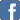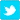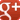# Fun With Numbers

I have been thinking about bases other than decimal (such as binary) and quadratic equations. These are normally treated as separate topics, but there are overlaps, and if you think it through, you reach some surprising conclusions.
Whether there will ever be any practical application of this is doubtful, so only read on if you have quarter of an hour to spare. Here goes...
Bases other than decimal
We use decimal numbers (base ten) all the time, but I hope that most people are also familiar with the concept of binary numbers (base 2). They used to teach it at school (heck knows why, but it was fun).
If you see the number "11" you assume that it means "eleven", but if you are told this is using binary notation, it means "three". Instead of columns for hundreds, tens and units, you have columns for fours, twos and units.
You can use any base you like, the computer chaps like using hexadecimal (base sixteen), They use digits up to nine and use A for "ten", B for "eleven" and so on up to F for "fifteen" (the highest digit is always one less than the base), The columns are for 256s (16 squareds), sixteens and units. So if "1A3" is a number in hexadecimal, its decimal equivalent is 419.
It's fun writing down numbers in other bases and trying to add, subtract, multiply and divide. It really messes with your mind, if you like that sort of thing.
If you are asked to solve something like "x^2 + 2x + 3 = 27" for x, it is quite a faff because you have to use one of the techniques for solving it, and you have to concentrate on not getting confused between x-squareds and x's.
My first breakthrough was to realize that we are all actually very familiar with this format/concept... because that's how we write down numbers.
The number "111" (in base ten) means 1 x 100 (ten squared) + 1 x 10 + 1. = 111. Easy. You can also imagine it as a quadratic equation x^2 + x + 1 = 111 where x = ten.
The number "111" (in base 2) = "7" (in base ten). If you know we're using binary, that's also simple enough. It's a two squared, plus a two, plus a unit.
Putting two and two together... to make five
Some maths puzzles give you a calculation that looks odd, such as "12 x 13 = 222" and your challenge is to work out what base this is in. By trial and error, you can work out that it is in base four*. "12" means 4 + 2 and "13" means 4 + 3, the answer is 42 (in decimal terms), which in base four is expressed as "222" (2 x 16 + 2 x 4 + 2).
So... when you are solving a quadratic equation where the signs before the x-squareds and the x's are positive, solving for x is the same as asking what base it is in!
If I tell you that "2x^2 + 2x + 2 = 42", you can turn that into two very similar questions.
1. You can turn it into "222 in a non-decimal base = 42 in base ten" and ask "Which base is 222 in?", and somehow you work out it is in base 4.
Or...
2. You can solve for x. If you've been following so far, you know that one of the answers must be four... but negative five is the other valid answer.
So there actually two possible answers to the first question - it could be base four or it could be base negative five.
It is here that my train of thought grinds to a halt. It would be fun to argue the toss with a maths teacher whether he would accept "base negative five" as a valid answer to question 1*.
Apart from that I see no practical use to this concept - could there ever conceivably be a situation in which it is handy to use a negative base, in the same way as the computer chaps find hexadecimal and binary useful?
* Of course, another answer to the problem "in which base is 13 X 14 = 222 a valid equation?" is "negative one" if you multiply up and solve it using the quadratic equation approach, but while I accept the existence of negative bases, there can be no base one, because the highest digit you can use is one less than the base, in this case zero.